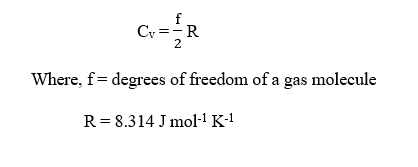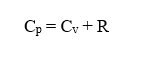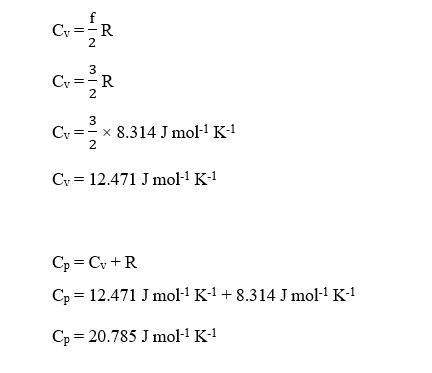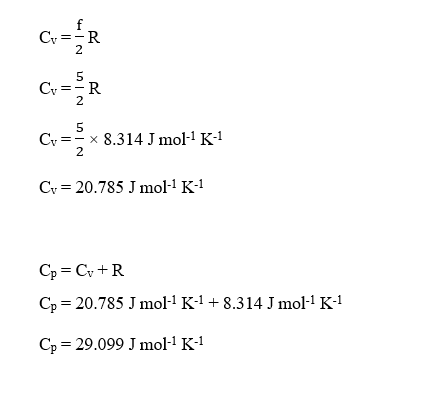# Calculate the theoretical value of C_p (constant pressure heat capacity)/ C_v (constant volume heat capacity) for the following gases: Ar, CO_2, and N_2

Question
1 views

Calculate the theoretical value of C_p (constant pressure heat capacity)/ C_v (constant volume heat capacity) for the following gases: Ar, CO_2, and N_2

check_circle

Step 1

The molar specific heat capacity of a gas at constant volume (Cv) is the amount of heat required to raise the temperature of 1 mol of the gas by 1 °C at the constant volume.

The specific heat at constant volume is given as :The molar specific heat of a gas at constant pressure (Cp) is the amount of heat required to raise the temperature of 1 mol of the gas by 1 °C at the constant pressure.

The specific heat at constant pressure (Cp) is greater than that at constant volume (Cv). The relationship between Cv and Cp is given as :For monoatomic gas :

The degrees of freedom, f = 3 (3 translational motion only)

For diatomic gas :

The degrees of freedom, f = 5 (3 translational + 2 rotational)

For triatomic gas (linear molecule) :

The degrees of freedom, f = 5 (3 translational + 2 rotational)

For triatomic gas (non-linear molecule) :

The degrees of freedom, f = 6 (3 translational + 3 rotational)

Step 2

The given gases are

Ar :

This is a monoatomic gas.

Degree of freedom, f = 3

The theoretical value of Cv and Cp can be calculated as :Step 3

CO2 :

This is a linear triatomic gas.

Degree of freedom, f = 5

The theoretical value of Cv and Cp can be calculated as :...

### Want to see the full answer?

See Solution

#### Want to see this answer and more?

Solutions are written by subject experts who are available 24/7. Questions are typically answered within 1 hour.*

See Solution
*Response times may vary by subject and question.
Tagged in